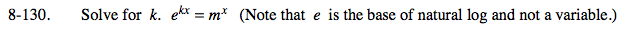### Home > PC > Chapter 8 > Lesson 8.2.4 > Problem8-130

8-130.

Solve for k. ekx = mx (Note that e is the base of natural log and not a variable.) Homework Help ✎Take the natural log of both sides and bring the exponents down in front.

ekx = mx
(kx)ln(e) = (x)ln(m)
(kx) = (x)ln(m)
(k) = ln(m)